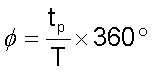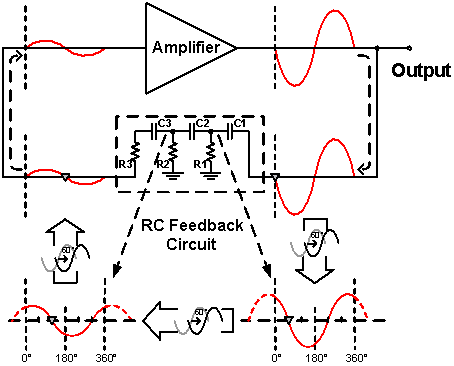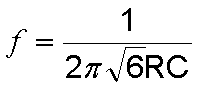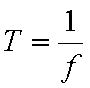# Oscillators

Analog Lab > Oscillators

An Oscillator is a circuit that produces a Periodic Waveform with only DC Power Supply. Depending on the type of Oscillator, the Output Waveform can be sinusoidal or non-sinusoidal. This Exhibit is a Sinusoidal Oscillator with Feedback.

Feedback is a process in which part of the Output Signal is returned to its Input in order to regulate its further Output, Figure 1. A Feedback Circuit can be built by RC or LC components.Figure 1: Block diagram of a Feedback System

To be exact, this Exhibit is a Phase Shift Oscillator with RC Feedback. Phase Shift is the difference in angular degrees between two signals with the same Frequency. In Figure 2, waveform 2 lags waveform 1 by the time tp, to express this time difference in angular degree, divide the time difference tp by the waveform period T and multiply the result by 360°. In Figure 2, the Phase Shift is 60°.Figure 2: Phase shift expressed in angular degrees

Oscillator requires a Phase Shift of 360° to operate probably. In Figure 3, the Amplifier itself provides a Phase Shift of 180°, and each RC circuit is designed to provide a Phase Shift of 60°.Figure 3: Block diagram of the Phase Shift Oscillator with RC Feedback

The Oscillation Frequency ( f ) of this Oscillator is stated in the following formula where Resistance R = R1 = R2 = R3 and Capacitance C = C1 = C2 = C3:Frequency f is the reciprocal of Period T :Analog Lab > Oscillators